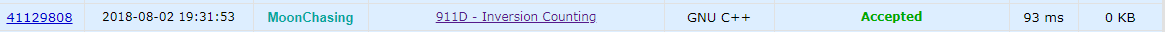## D. Inversion Counting

time limit per test: 2 seconds
memory limit per test: 256 megabytes
input: standard input
output: standard output

## Description

A permutation of size n is an array of size n such that each integer from 1 to n occurs exactly once in this array. An inversion in a permutation p is a pair of indices (i, j) such that i > j and a**i < *a**j*. For example, a permutation [4, 1, 3, 2] contains 4 inversions: (2, 1), (3, 1), (4, 1), (4, 3).

You are given a permutation a of size n and m queries to it. Each query is represented by two indices l and r denoting that you have to reverse the segment [l, r] of the permutation. For example, if a = [1, 2, 3, 4] and a query l = 2, r = 4 is applied, then the resulting permutation is [1, 4, 3, 2].

After each query you have to determine whether the number of inversions is odd or even.

### Input

The first line contains one integer n (1 ≤ n ≤ 1500) — the size of the permutation.

The second line contains n integers a1, a2, …, a**n (1 ≤ a**i ≤ n) — the elements of the permutation. These integers are pairwise distinct.

The third line contains one integer m (1 ≤ m ≤ 2·105) — the number of queries to process.

Then m lines follow, i-th line containing two integers l**i, r**i (1 ≤ l**i ≤ r**i ≤ n) denoting that i-th query is to reverse a segment [l**i, r**i] of the permutation. All queries are performed one after another.

### Output

Print m lines. i-th of them must be equal to odd if the number of inversions in the permutation after i-th query is odd, and even otherwise.

## Solution

### AC 代码# When Who Problem Lang Verdict Time Memory
41129808 2018-08-02 19:31:53 MoonChasing 911D - Inversion Counting GNU C++ Accepted 93 ms 0 KB
❤采之欲遗谁，所思在远道。❤